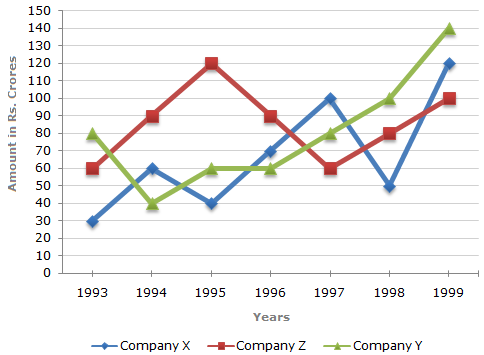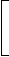# Data Interpretation - Line Charts - Discussion

Study the following line graph and answer the questions.

Exports from Three Companies Over the Years (in Rs. crore)2.

Average annual exports during the given period for Company Y is approximately what percent of the average annual exports for Company Z?

 [A]. 87.12% [B]. 89.64% [C]. 91.21% [D]. 93.33%

Explanation:

Analysis of the graph: From the graph it is clear that

1. The amount of exports of Company X (in crore Rs.) in the years 1993, 1994, 1995, 1996, 1997, 1998 and 1999 are 30, 60, 40, 70, 100, 50 and 120 respectively.

2. The amount of exports of Company Y (in crore Rs.) in the years 1993, 1994, 1995, 1996, 1997, 1998 and 1999 are 80, 40, 60, 60, 80, 100 and 140 respectively.

3. The amount of exports of Company Z (in crore Rs.) in the years 1993, 1994, 1995, 1996, 1997, 1998 and 1999 are 60, 90,, 120, 90, 60, 80 and 100 respectively.

Average annual exports (in Rs. crore) of Company Y during the given period

 = 1 x (80 + 40 + 60 + 60 + 80 + 100 + 140) = 560 = 80. 7 7

Average annual exports (in Rs. crore) of Company Z during the given period

 = 1 x (60 + 90 + 120 + 90 + 60 + 80 + 100) =600. 7 7Required percentage =80 x 100%93.33%.6007

 Aditya said: (Aug 12, 2010) Hi All, I'm preparing for CAT-2010. Can you please share tricks to solve Line Charts. Thanks n regards, Aditya

 Sasi said: (Feb 5, 2011) Follow the IndiaBIX line charts. I hope you will get different points.

 Hridya said: (Oct 26, 2011) I'm preparing CAT-2011. Please share the tricks to solve line charts & bar diagrms.

 Zakaria said: (Apr 29, 2012) No need to calculate average! because each company contain 7 years exports. total of Y=560 total of Z=600 so, percentage=560/600*100=93.33

 Haffy said: (Jul 26, 2013) Please help me on how to get the percentage without using calculator?

 Ashi said: (Apr 18, 2015) @Haffy. Keep practicing numbers have command on your multiples upto 25 that it. All the best.

 Suhasini said: (May 16, 2018) No need to find average directly, First, find percentage = 560*100/600 = 93.33.

 Janhvi said: (Jun 14, 2018) After calculating the average which formula used? Please tell me.

 Rishika said: (Oct 5, 2019) How to solve the problem in Very less time?